# Controlled power supply increases op amps' output-voltage range

Yakov Velikson

EDN

Increasing the output voltage of IC operational amplifiers usually involves adding high-voltage external transistors. The resulting circuit then requires correction to retain its operating characteristics. This correction is difficult, especially for precise amplifiers. This Design Idea presents an alternative: the use of a controlled power supply for the operational amplifier itself, which can increase the output voltage for many precise operational amplifiers without altering their operational characteristics. You can accomplish this task by connecting controlled transistors to the power supply of the amplifier. Resistor dividers that connect to the amplifier’s output and bipolar high voltages control these transistors (Reference 1). The simple circuits in Figure 1 present general methods of connecting the amplifier as an inverter or a follower to effect increased output voltage.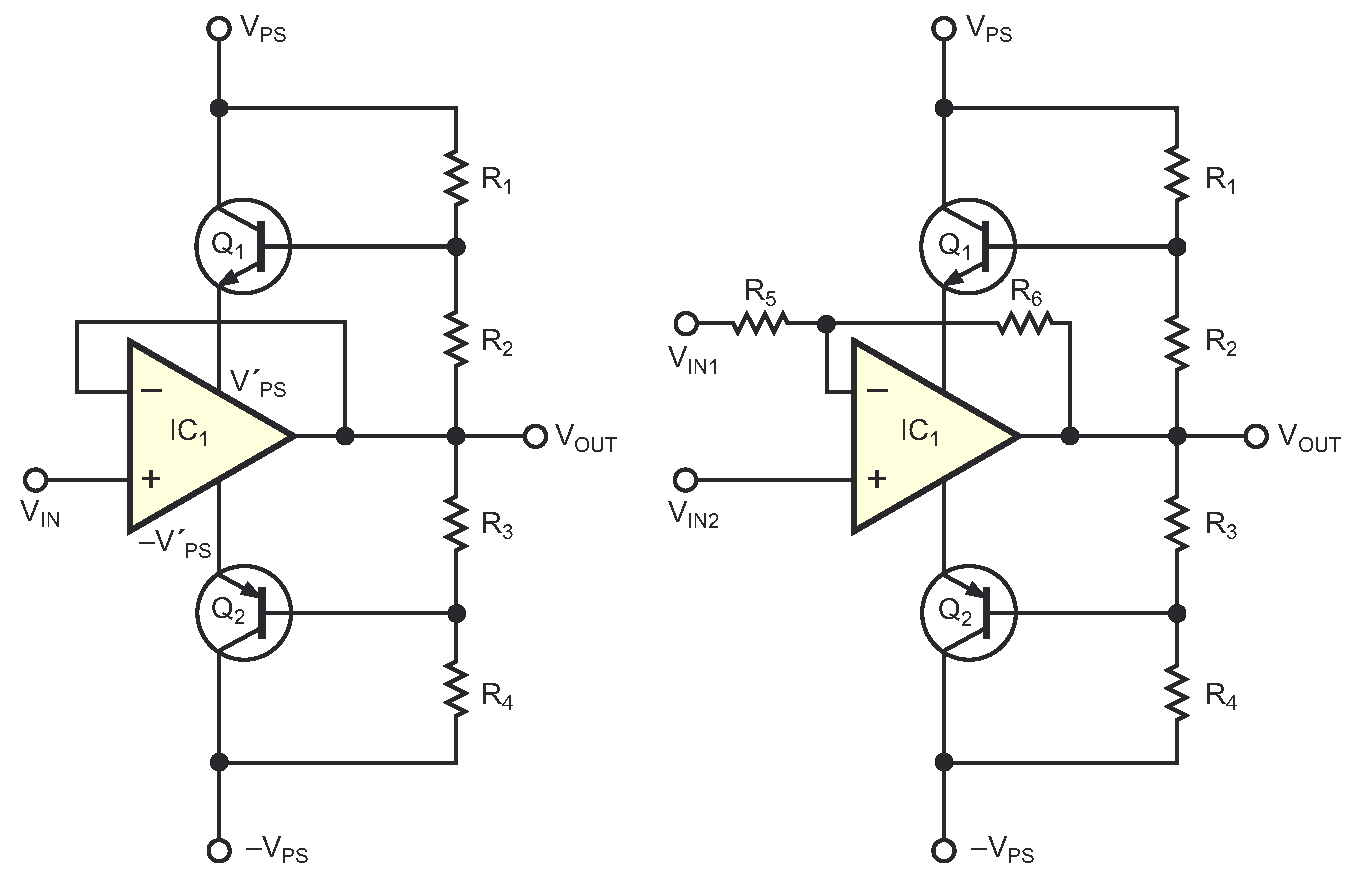Figure 1. These simple circuits present the general methods of connecting the amplifier as an inverter or a follower to effect increased output voltage.

Dividers with resistors R1, R2, R3, and R4 determine the scale of power supply V'PS and –V'PS for the amplifiers. If the output voltage ranges from ±22 V, resistor R1 = R2 = R3 = R4 = R, and VPS and –VPS are 28 V, then voltages V'PS and –V'PS fall in the following range, allowing for any additional loss: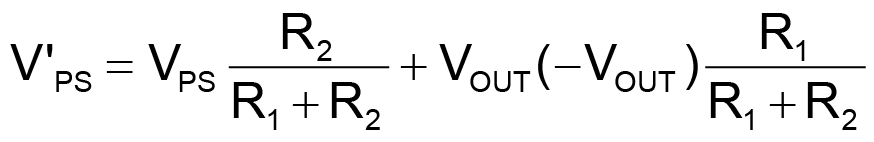and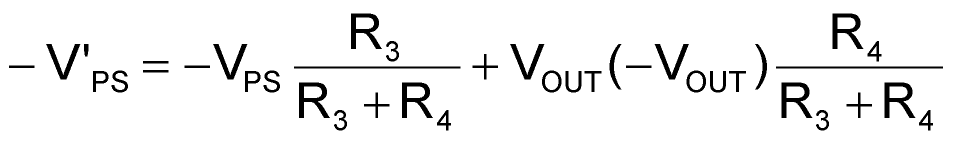or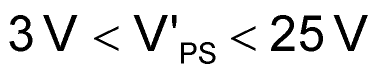and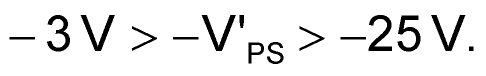However, power- supply circuits include transistors, which create junction resistance, affecting the amplifier’s operation.

You can use supporting amplifiers to reduce losses and increase the quality of the output voltage of the primary amplifier. The requirements for supporting amplifiers are simple. They should have power supplies with opposite polarity from and lower applied voltage than that of the main power supply. They should provide the necessary power to the primary amplifier, and their frequency range should be slightly higher than that of the primary amplifier. You can use supporting amplifiers to eliminate the transitional resistances of transistors in power-supply connections. Thus, these circuits offer flexibility across a range of amplifier configurations (references 2 and 3).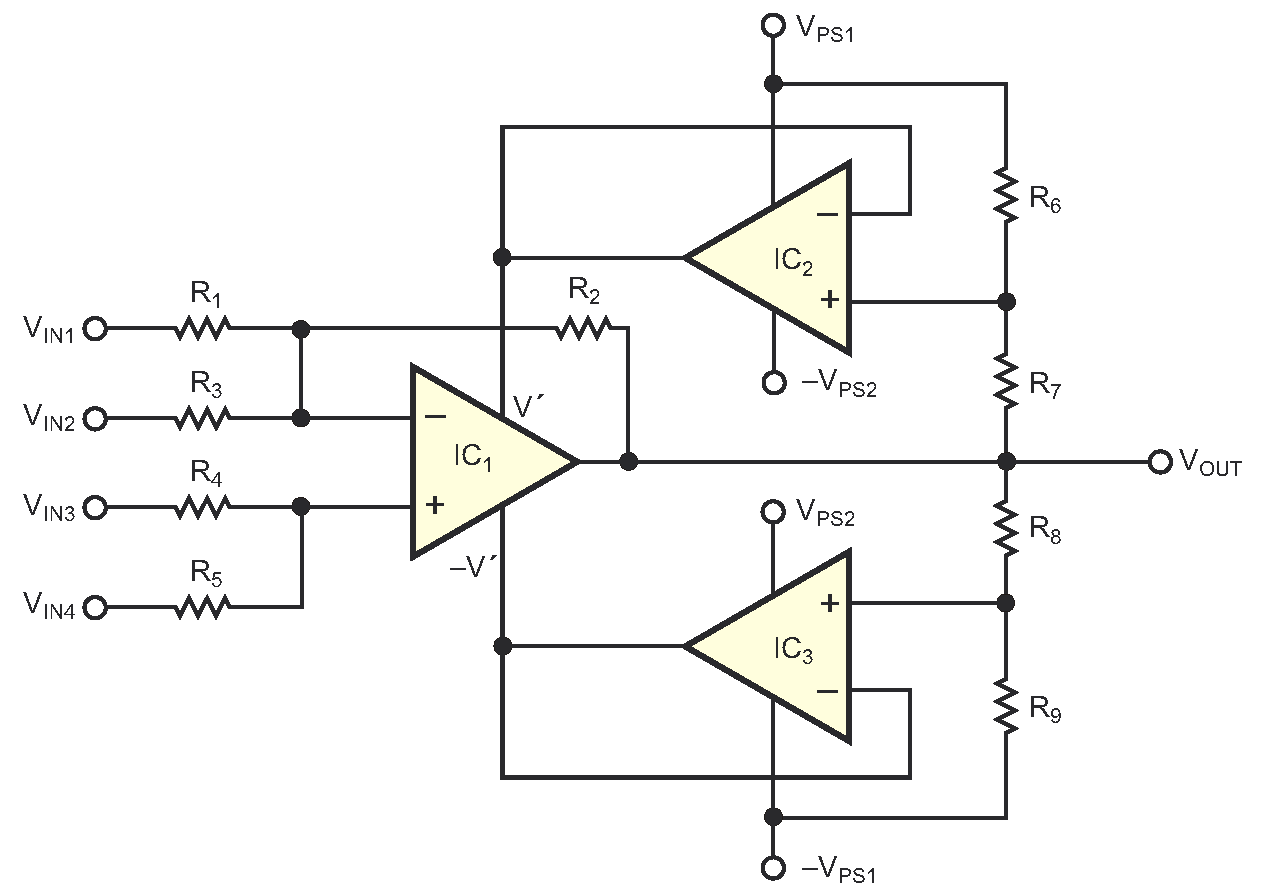Figure 2. Replacing the transistors with op amps reduces losses and increases the quality of the output voltage of the primary amplifier.

Figure 2 shows an example of how to connect supporting amplifiers as followers. You derive output voltages V'PS and –V'PS from resistor connections with the following equations: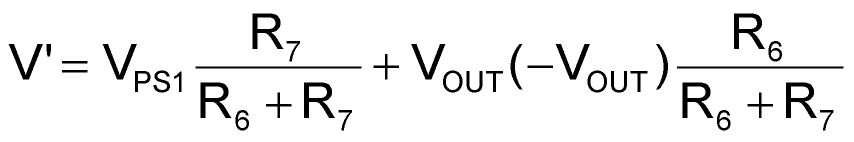and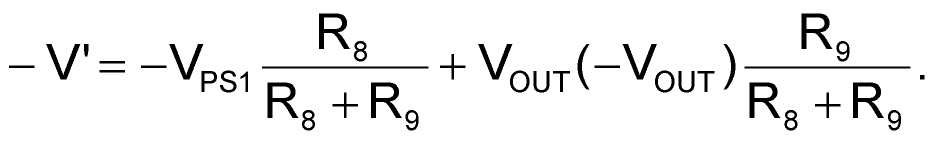If the supporting amplifiers have a power supply of 28 V for VPS1 and –2 V for –VPS2 for amplifier IC2, then –VPS1 = –28 V, VPS2 = 2 V for amplifier IC3, and the output voltage of amplifier IC1, VOUT, is 24 V or –24 V. Also, R6 = R7 = R8 = R9 = R, such that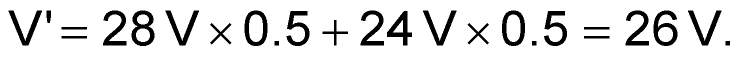Further,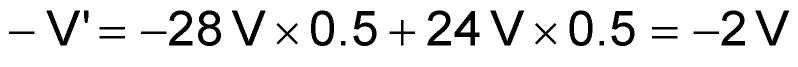for VOUTMAX = 24 V.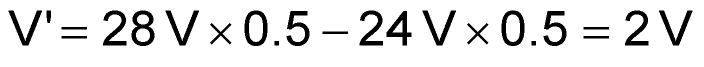andfor VOUTMIN = –24 V.

You can achieve the greatest voltage range by using separate power supplies – one for the normal voltages of the amplifier and one for the regulated part of the output voltage (Figure 3).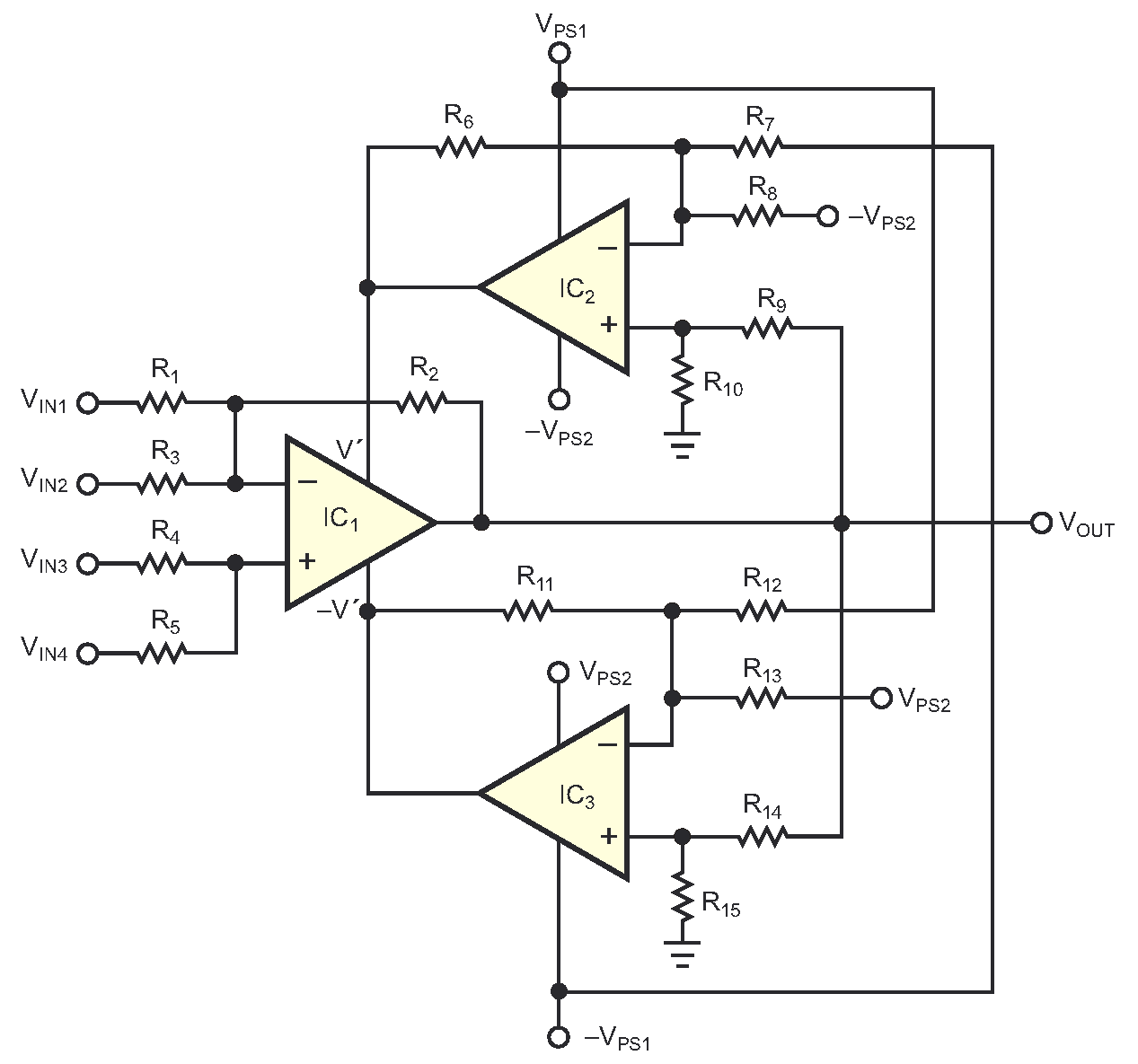Figure 3. You can achieve the greatest voltage range by using separate power supplies – one for the normal voltages of the amplifier and one for the regulated part of the output voltage.

IC1 is the primary amplifier. Supporting amplifiers IC2 and IC3 have asymmetrical power supplies. You could use many types of amplifiers in this circuit, but modern operational amplifiers may be preferable because they allow the use of the complete range of the power supply and because they handle rail-to-rail input and output. In this circuit, VPS1 = 28 V, –VPS1 = 28 V, VPS2 = 2 V, and –VPS2 = 2 V.

The voltages of the primary amplifier are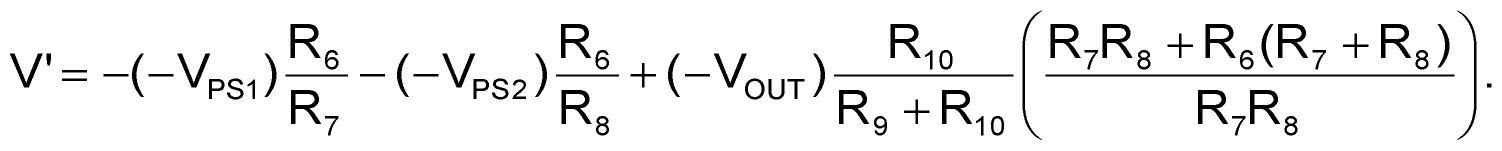Further,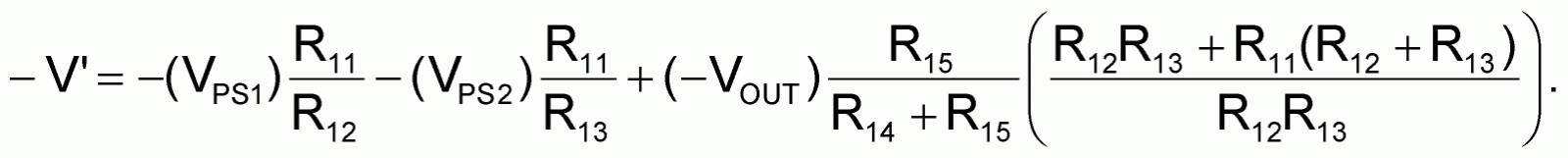Set R6 = R10 = R11 = R15 = R, R7 = R8 = R12 = R13 = 2R, and R9 = R14 = 3R, such that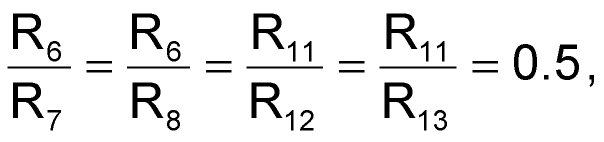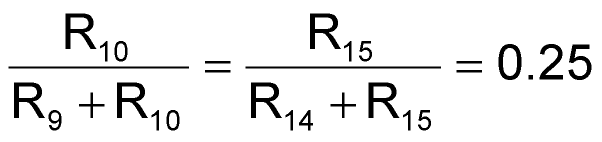and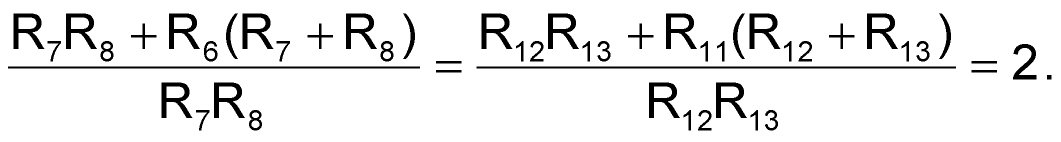Then, substitute these values into the amplifier voltages yields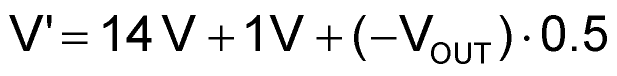and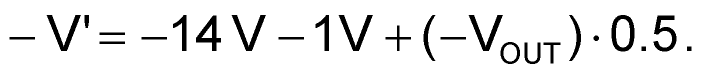Minimum and maximum values for each power supply are 1.5 V ⩽ V ⩽ 28.5 V, and –1.5 V ⩾ –V ⩾ –28.5 V. The total voltage of the power supply has a limit of 30 V, ranging from 1.5 to 28.5 V and from –1.5 to –28.5 V. This range permits an increase of the output voltage of the primary amplifier by ±27 V.

## References

1. Velikson Yakov, Electronics, Information Book, pg 138, Energoatomiztum, St Petersburg, Russia, 1996.
2. Velikson Yakov, “Device for recurrence of voltage,” Bulletin of Izobreteny (copyrights and patents), No. 3, 1991.
3. Velikson Yakov, A Murigin, and V Uchno, “The multichannel conversion of a revolving sine-cosine resolver to code,” Bulletin of Izobreteny (copyrights and patents), No. 44, 1988.

EDN